PRINTABLE FOR KIDS

XII (12) HSC

XI (11) FYJC
X (10) SSC

### Financial Planning Class 9th Mathematics Part I MHB Solution

##### Class 9th Mathematics Part I MHB Solution

###### Practice Set 6.1
Question 1.

Alka spends 90% of the money that she receives every month and saves Rs. 120. How much money does she get monthly?

Method 1: Let the total money be = Rs. x

Now money she spends = 90% of x =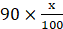The money she saves = 120

Now

Money spent + Money saved = Total Money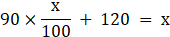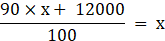90x + 12000 = 100x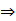100x – 90x = 1200010x = 12000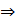x = 1200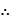She earns Rs 1200 .

Method 2:
percentage of spending = 90%

hence the percentage of savings = 100 - 90%
= 10%
If 10% savings is Rs.120,

Total money received =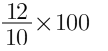= Rs.1200

Question 2.

Sumit borrowed a capital of Rs. 50,000 to start his food products business. In the first year he suffered a loss of 20%. He invested the remaining capital in a new sweets business and made a profit of 5%. How much was his profit or loss computed on his original capital?

Capital borrowed by Sumit = Rs 50,000

Now, Loss in first year = 20% of Rs 50,000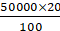= Rs 10,000

∴ Remaining capital = Rs 50,000 – Rs 10,000

= Rs 40,000

Then,

He invested remaining capital in sweet business and had a profit of 5%.

∴ Profit amount = 5% of 40000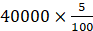= Rs 2000

He occurred a profit of Rs 2000.

∴ The capital will be Rs 40000 + Rs 2000

= Rs 42,000.

Now,

Original capital = Rs 50,000

New capital after 2 years = Rs 42,000

∴ Loss% =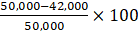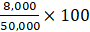= 16%

Hence, his capital after 2 years = Rs 42,000 & he suffered a loss of 16% on the initial capital.

Question 3.

Nikhil spent 5% of his monthly income on his children's education, invested 14% in shares, deposited 3% in a bank and used 40% for his daily expenses. He was left with a balance of Rs. 19,000. What was his income that month?

Let the monthly salary of Nikhil = Rs x

The following table gives the expenditure of Nikhil: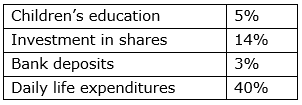∴ Total expenditure = 5% + 14% + 3% + 40%

= 62%

Now, remaining salary = 100% - 62% of total salary

= 38% of total salary

= Rs 19,000 ( given)

∴ 38% of x = Rs 19,000

⇒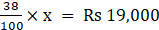⇒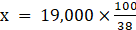⇒ x = Rs 50,000Hence, the monthly income of Nikhil = Rs 50,000.

Question 4.

Mr. Sayyad kept Rs. 40,000 in a bank at 8% compound interest for 2 years. Mr. Fernandes invested Rs. 1,20,000 in a mutual fund for 2 years. After 2 years, Mr. Fernandes got Rs. 1,92,000. Whose investment turned out to be more profitable?

Principal amount, P = 40,000

Rate, r = 8%

Time period = n = 2year

Compound Interest (C.I) =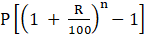C.I =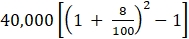= 40,000(1.664-1)

= 40,000(0.664)

= Rs. 6656

% profit(p) =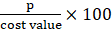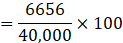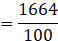= 16.64%

For Fernandes,

Principal rate, P = Rs. 1,20,000

Time period, n = 2yr

Principal amount, A = Rs. 1,92,000

Profit = 1,92,000 – 1,20,000 = Rs. 72,000.

%Profit (p) =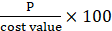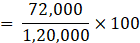= 60%.

Hence, Frenandes mutual fund is found to be profitable.

Question 5.

Sameera spent 90% of her income and donated 3% for socially useful causes. If she left with Rs. 1750 at the end of the month, what was her actual income?

Given,

Let Sameera’s income be Rs x.

Then, amount she spends = 90% of x

Donations by Sameera = 3% of x

Remaining amount = 100 – (90 + 3)% of x

And, the remaining amount = Rs. 1750

Here, 7% of x = Rs 1750

⇒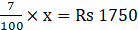⇒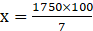⇒ x = Rs 25,000

∴ her monthly income is Rs. 25,000.

###### Practice Set 6.2
Question 1.

Observe the table given below. Check and decide, whether the individuals have to pay income tax.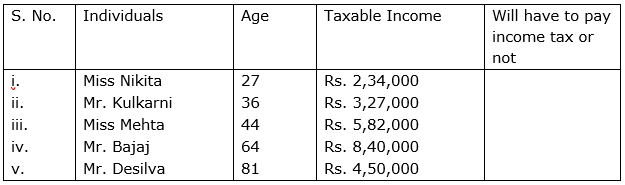The tax rates for a year budget are shown below are given in the three tables:

Table I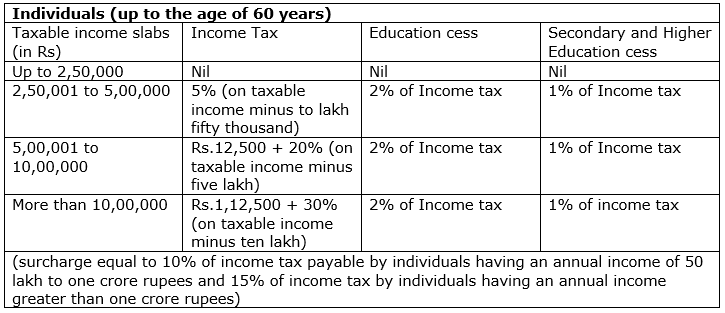Table II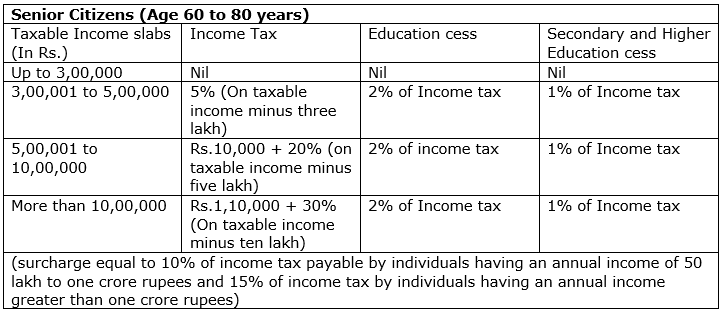Table III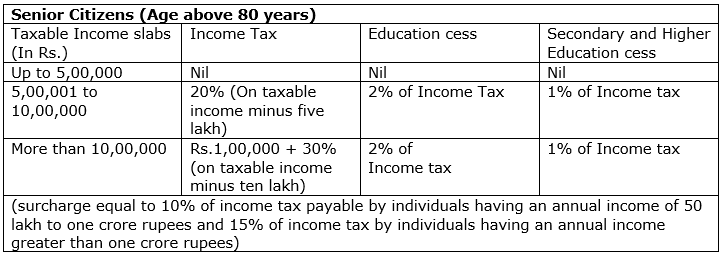i) No,

According to 1st table because Annual Income is less than 2.5lacs.

Hence, she is not eligible for paying the income tax.

ii) Yes, because Mr. Kulkarni’s salary lies between 2.5 l to 5 l. Hence, he is eligible for paying tax.

iii)Yes, because the income is greater than 2.5 l (from table I)

iv)Yes, because the income is greater than 2.5 l.

v)No, because (table 3)

Question 2.

Mr. Kartarsingh (age 48 years) works in a private company. His monthly income after deduction of allowances is Rs. 42,000 and every month he contributes Rs. 3000 to GPF. He has also bought Rs. 15,000 worth of NSC (National Savings Certificate) and donated Rs. 12,000 to the PM's Relief Fund. Compute his income tax.

According to 1st table,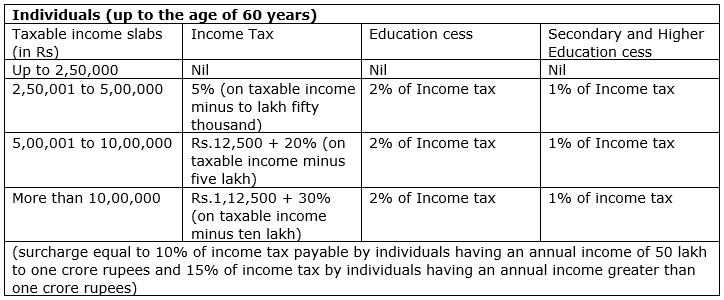His monthly income = Rs 42,000

⇒ Annual Salary = 12 × Rs 42,000

= Rs. 5,04,000

Now, following table shows his investments: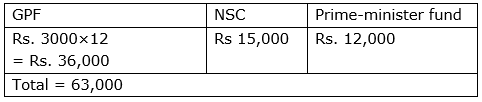Taxable salary = Rs. 5,04,000 – (Rs. 63,000)

= Rs. 4,41,000

It comes into table 1 and income tax slab 2.

5%(taxable minus 2.5lacs) =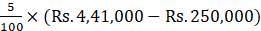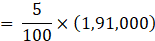= Rs.9550

∴ Income tax = Rs.9550

Primary cess = 2% of I.T.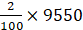= Rs.191

Secondary cess = 1% of I.T.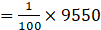= Rs.95.5

Total income tax = Rs.9550 + Rs.191 + Rs.95.5

= Rs.9836.5

###### Problem Set 6
Question 1.

Write the correct alternative answer for each of the following questions.

For different types of investments what is the maximum permissible amount under section 80C of income tax?
A. 1,50,000 rupees

B. 2,50,000 rupees

C. 1,00,000 rupees

D. 2,00,000 rupees

The maximum amount of deduction that can be claimed under Section 80C of the Income Tax Act is Rs.1.5 lakh.

Question 2.

Write the correct alternative answer for each of the following questions.

A person has earned his income during the financial year 2017-18. Then his assessment year is ....
A. 2016-17

B. 2018-19

C. 2017-18

D. 2015-16

The period in which the income is earned is called the financial year. And, the income tax are filed in the next year of the financial year. It is called the assessment year.

∴ if he earned his income in 2017-18, his assessment year will be 2018-19.

Question 3.

Mr. Shekhar spends 60% of his income. From the balance he donates Rs. 300 to an orphanage. He is then left with Rs. 3,200. What is his income?

Mr. Shekar’s income = Rs x

Amount spent = 60% of x =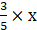Remaining balance =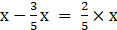Donation to an orphanage = Rs.300

Remaining balance =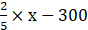= Rs.3200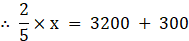⇒ 2x =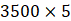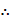x = Rs.8750

Income is Rs. 8750.

Question 4.

Mr. Hiralal invested Rs. 2,15,000 in a Mutual Fund. He got Rs. 3,05,000 after 2 years. Mr. Ramniklal invested Rs. 1,40,000 at 8% compound interest for 2 years in a bank. Find out the percent gain of each of them. Whose investment was more profitable?

According to question,

For Ramnikal,

Principal amount = Rs. 1,40,000

Rate, r = 8%

Time period, n = 2years

Compound interest (CI) =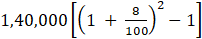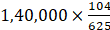= Rs.23296

% gain =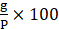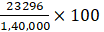= 16.64%

For Hiralal,

Principal amount = Rs. 2,15,000

Amount gained = Rs. 3,05,000

Time period, n = 2year

Profit/gain = A - P

= 3,05,000 – 2,15,000

= Rs. 90,000

% gain =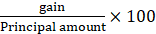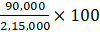= 41.86%

Mr. Hiralal investment is more profitable.

Question 5.

At the start of a year there were Rs. 24,000 in a savings account. After adding Rs. 56,000 to this the entire amount was invested in the bank at 7.5% compound interest. What will be the total amount after 3 years?

Balance at the start of the year = Rs. 24000

Total = Rs. 80,000

Interest rate = 7.5% per annum

Period of accumulation = 3yr

Formula,

A =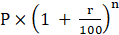Amount of maturity after 3 year,

= Rs.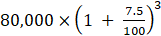= Rs. 80,000×1.075×1.075×1.075

= Rs. 99,383.75

Question 6.

Mr. Manohar gave 20% part of his income to his elder son and 30% part to his younger son. He gave 10% of the balance as donation to a school. He still had Rs. 1,80,000 for himself. What was Mr. Manohar's income?

Mr. Manohar’s income = A

Amount given to elder son = 20% of A =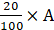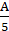Amount given to younger son = 30% of A =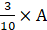Balance = 100% - 30% -20%

= 50%of A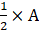Donation given to school =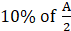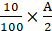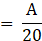Balance =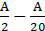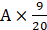⇒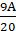= Rs.180000

A = Rs.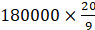= Rs. 400,000

⇒ Income is Rs 4,00,000.

Question 7.

Kailash used to spend 85% of his income. When his income increased by 36% his expenses also increased by 40% of his earlier expenses. How much percentage of his earning he saves now?

Let Kailash’s salary = Rs. 100

Old spend = 100×85% = 85

New salary = 136%of 100 = Rs.136

New expenditure =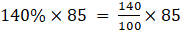= Rs.116

Save = Rs. 136 – Rs. 119

= Rs.17

%S =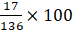= 12.5%

Question 8.

Total income of Ramesh, Suresh and Preeti is 8,07,000 rupees. The percentages of their expenses are 75%, 80% and 90% respectively. If the ratio of their savings is 16 : 17 : 12, then find the annual saving of each of them.

Let their savings be 16x,17x,12x

Saving = income – expenditures

Saving% = 100 – expenditure%

Hence saving are 25% ,20% , 10% respectively,

Saving % =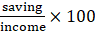Their incomes are,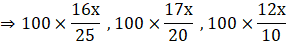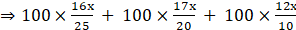= Rs.807000

Then x = Rs. 3000

Hence saving are Rs. 48,000 , Rs. 51,000 , Rs. 36,000

Question 9.

Compute the income tax payable by following individuals.

i. Mr. Kadam who is 35 years old and has a taxable income of Rs. 13,35,000.

ii. Mr. Khan is 65 years of age and his taxable income is Rs. 4,50,000.

iii. Miss Varsha (Age 26 years) has a taxable income of Rs. 2,30,000.

i) In case of Mr.kadam who is 35 years and taxable income is 13,35,000

•up to 250000 - No tax (0 %)

•2,50,000 to 5,00,000 - 12,500 (2,50,000 x 5%)

•5,00,000 to 10,00,000 - 1,00,000 (5,00,000 x 20%)

•remaining 3,35,000 - 1,00,500 (3,35,000 x 30%)

Income Tax is 2,13,000 + Education cess 3% on 2,13,000

Total tax is 2,19,390

(ii) in the case of Mr. Khan who is 65 yrs and 4,50,000 income taxable

upto 300000 - No tax ( 0 % )

remaining 150000 - 7500 ( 150000 x 5%)

Income Tax is 7500 + Education Cess 3% of 7500

Total Tax is 7725

(iii) In the case of Miss Varsha who is 26 yrs and 2,30,000 taxable income.

upto 2,50,000 income - no tax

Total tax - NIL

## PDF FILE TO YOUR EMAIL IMMEDIATELY PURCHASE NOTES & PAPER SOLUTION. @ Rs. 50/- each (GST extra)

SUBJECTS

HINDI ENTIRE PAPER SOLUTION

MARATHI PAPER SOLUTION
SSC MATHS I PAPER SOLUTION
SSC MATHS II PAPER SOLUTION
SSC SCIENCE I PAPER SOLUTION
SSC SCIENCE II PAPER SOLUTION
SSC ENGLISH PAPER SOLUTION
SSC & HSC ENGLISH WRITING SKILL
HSC ACCOUNTS NOTES
HSC OCM NOTES
HSC ECONOMICS NOTES
HSC SECRETARIAL PRACTICE NOTES

2019 Board Paper Solution

HSC ENGLISH SET A 2019 21st February, 2019

HSC ENGLISH SET B 2019 21st February, 2019

HSC ENGLISH SET C 2019 21st February, 2019

HSC ENGLISH SET D 2019 21st February, 2019

SECRETARIAL PRACTICE (S.P) 2019 25th February, 2019

HSC XII PHYSICS 2019 25th February, 2019

CHEMISTRY XII HSC SOLUTION 27th, February, 2019

OCM PAPER SOLUTION 2019 27th, February, 2019

HSC MATHS PAPER SOLUTION COMMERCE, 2nd March, 2019

HSC MATHS PAPER SOLUTION SCIENCE 2nd, March, 2019

SSC ENGLISH STD 10 5TH MARCH, 2019.

HSC XII ACCOUNTS 2019 6th March, 2019

HSC XII BIOLOGY 2019 6TH March, 2019

HSC XII ECONOMICS 9Th March 2019

SSC Maths I March 2019 Solution 10th Standard11th, March, 2019

SSC MATHS II MARCH 2019 SOLUTION 10TH STD.13th March, 2019

SSC SCIENCE I MARCH 2019 SOLUTION 10TH STD. 15th March, 2019.

SSC SCIENCE II MARCH 2019 SOLUTION 10TH STD. 18th March, 2019.

SSC SOCIAL SCIENCE I MARCH 2019 SOLUTION20th March, 2019

SSC SOCIAL SCIENCE II MARCH 2019 SOLUTION, 22nd March, 2019

XII CBSE - BOARD - MARCH - 2019 ENGLISH - QP + SOLUTIONS, 2nd March, 2019

HSC Maharashtra Board Papers 2020

(Std 12th English Medium)

HSC ECONOMICS MARCH 2020

HSC OCM MARCH 2020

HSC ACCOUNTS MARCH 2020

HSC S.P. MARCH 2020

HSC ENGLISH MARCH 2020

HSC HINDI MARCH 2020

HSC MARATHI MARCH 2020

HSC MATHS MARCH 2020

SSC Maharashtra Board Papers 2020

(Std 10th English Medium)

English MARCH 2020

HindI MARCH 2020

Hindi (Composite) MARCH 2020

Marathi MARCH 2020

Mathematics (Paper 1) MARCH 2020

Mathematics (Paper 2) MARCH 2020

Sanskrit MARCH 2020

Important-formula

THANKS